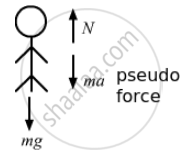# A Person is Standing on a Weighing Machine Placed on the Floor of an Elevator. the Elevator Starts Going up with Some Acceleration, Moves with Uniform Velocity for a While and F - Physics

Sum

A person is standing on a weighing machine placed on the floor of an elevator. The elevator starts going up with some acceleration, moves with uniform velocity for a while and finally decelerates to stop. The maximum and the minimum weights recorded are 72 kg and 60 kg, respectively. Assuming that the magnitudes of acceleration and deceleration are the same, find (a) the true weight of the person and (b) the magnitude of the acceleration. Take g = 9.9 m/s2.

#### SolutionMaximum weight will be recorded when the elevator accelerates upwards.
Let N be the normal reaction on the person by the weighing machine.
So, from the free-body diagram of the person,
$N = mg + ma$    ...(1)
This is maximum weight, N = 72 × 9.9 N
When decelerating upwards, minimum weight will be recorded.
$N' = mg + m\left( - a \right)$   ...(2)
This is minimum weight, N' = 60 × 9.9 N
From equations (1) and (2), we have:
2 mg = 1306.8
$\Rightarrow m = \frac{1306 . 8}{2 \times 9 . 9} = 66 kg$
So, the true mass of the man is 66 kg.
And true weight = 66 $\times$ 9.9 = 653.4 N
(b) Using equation (1) to find the acceleration, we get:
mg + ma = 72 × 9.9
$\Rightarrow a = \frac{72 \times 9 . 9 - 66 \times 9 . 9}{66} = \frac{9 . 9 \times 6}{66} = \frac{9 . 9}{11}$
$\Rightarrow a = 0 . 9 m/ s^2$

Concept: Newton’s Second Law of Motion
Is there an error in this question or solution?

#### APPEARS IN

HC Verma Class 11, Class 12 Concepts of Physics Vol. 1
Chapter 5 Newton's Laws of Motion
Q 15 | Page 80